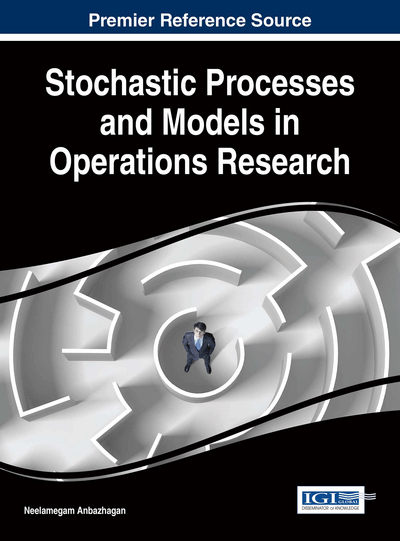On Sequential Decision Problems with Constant Costs of Observation

Sören Christensen (Chalmers University of Technology, Sweden) and Albrecht Irle (University of Kiel, Germany)
DOI: 10.4018/978-1-5225-0044-5.ch013

Abstract

We present a solution technique for optimal stopping problems with constant costs of observation in a diffusion setting. Such problems arise naturally, e.g., in Wald's type sequential decision problems and the Portfolio optimization model by Morton and Pliska. The main result is that the treatment of such problem boils down to the determination of the maximum points of a class of explicitly given functions. The findings are illustrated by a variety of examples and generalized to random costs of observation.
Chapter Preview
Top

Introduction

Problems of optimal stopping arise in a variety of different fields. They appear, for example, in mathematical statistics, derivative pricing, portfolio optimization, stochastic control theory, and in the general theory of probability and its connection to problems in analysis. Hence solutions to these problems are important both for theory and applications. Early textbook treatments are Chow et al. (1971) for the discrete case and Shiryaev (1978) for Markovian problems; a recent monograph is Peskir and Shiryaev (2006).

In this chapter, we consider optimal stopping problems for diffusion processes. This class of problems is studied very detailed for the case that a discounting factor is present, which is the case for many problems in Mathematical Finance. In other fields of application, a different cost structure is more natural, namely linear costs of observation. I.e. problems of the form

are of interest, starting with the seminal work of Wald and Wolfowitz (1950) in sequential statistics. Nowadays, this kind of problem is known to arise in many more fields, such as portfolio optimization, see Morton and Pliska (1995) and the work based on it. However, it is interesting to see that also the literature on sequential statistics with linear costs of observation is still growing, see, e.g., Gapeev and Shiryaev (2011) and Zhitlukhin and Shiryaev (2011).

for continuousand. Although this class of problems is very relevant, general solution techniques are not very well-known, but see Irle and Paulsen (2004) and Christensen (in press) for the case of general underlying Markov processes. The aim of this chapter is to describe a new method for the treatment of this class of problems, that leads to explicit solutions. It is based on the following observation:

then it holds that(1)and if the class of stopping times is rich enough, thenis in the optimal stopping set, i.e.

Complete Chapter List

Search this Book:
Reset